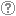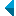# Iskanje po repozitorijuIskalni niz: išči po NaslovAvtorOpisKljučne besedePolno besediloLeto izida INALIIN NE išči po NaslovAvtorOpisKljučne besedePolno besediloLeto izida INALIIN NE išči po NaslovAvtorOpisKljučne besedePolno besediloLeto izida INALIIN NE išči po NaslovAvtorOpisKljučne besedePolno besediloLeto izida Vrsta gradiva: Vse vrste gradiv Habilitacijsko delo (m4) Višješolska diplomska naloga (m6) Specialistično delo (m3) Diplomsko delo * (dip) Magistrsko delo * (mag) Doktorska disertacija * (dok) Raziskovalni podatki ali korpus (data) * po starem in bolonjskem študiju Jezik: Vsi jezikiSlovenski jezikAngleški jezikNemški jezikHrvaški jezikSrbski jezikBolgarski jezikBosanski jezikČeški jezikFinski jezikFrancoski jezikItalijanski jezikJaponski jezikLitvanski jezikMadžarski jezikNemški jezik (Avstrija)Norveški jezikPoljski jezikRuski jezikSlovaški jezikSrbski jezik (cirilica)Španski jezikŠvedski jezikTurški jezikNeznan jezik Išči po: RUP    FAMNIT - Fakulteta za matematiko, naravoslovje in informacijske tehnologije    FHŠ - Fakulteta za humanistične študije    FM - Fakulteta za management    FTŠ Turistica - Fakulteta za turistične študije - Turistica    FVZ - Fakulteta za vede o zdravju    IAM - Inštitut Andrej Marušič    PEF - Pedagoška fakulteta    UPR - Univerza na Primorskem    ZUP - Založba Univerze na PrimorskemCOBISS    Fakulteta za humanistične študije, Koper    Fakulteta za management Koper in Pedagoška fakulteta Koper    Fakulteta za vede o zdravju, Izola    Knjižnica za tehniko, medicino in naravoslovje, Koper    Turistica, Portorož    Znanstveno-raziskovalno središče Koper Opcije: Prikaži samo zadetke s polnim besedilom Ponastavi

 1 - 10 / 4012341.On bipartite Q-polynomial distance-regular graphs with c [sub] 2 [equal] 1Štefko Miklavič, 2007, izvirni znanstveni članekOpis: Let ▫$\Gamma$▫ denote a bipartite ▫$Q$▫-polynomial distance-regular graph with diameter ▫$d \ge 3$▫, valency ▫$k \ge 3$▫ and intersection number ▫$c_2=1$▫. We show that ▫$\Gamma$▫ has a certain equitable partition of its vertex set which involves ▫$4d-4$▫ cells. We use this partition to show that the intersection numbers of ▫$\Gamma$▫ satisfy the following divisibility conditions: (I) ▫$c_{i+1}-1$▫ divides ▫$c_i(c_i-1)$▫ for ▫$2 \le i \le d-1$▫, and (II) ▫$b_{i-1}-1$▫ divides ▫$b_i(b_i-1)$▫ for ▫$1 \le i \le d-1$▫. Using these divisibility conditions we show that ▫$\Gamma$▫ does not exist if ▫$d=4$▫.Najdeno v: ključnih besedahPovzetek najdenega: ...mathematics, grah theory, distance-regular graphs, ▫$Q$▫-polynomial property, equitable... Ključne besede: mathematics, grah theory, distance-regular graphs, ▫$Q$▫-polynomial property, equitable partitionsObjavljeno: 15.10.2013; Ogledov: 3264; Prenosov: 35Polno besedilo (0,00 KB) 2.Isomorphism checking of I-graphsTomaž Pisanski, Boris Horvat, Arjana Žitnik, 2012, izvirni znanstveni članekOpis: We consider the class of ▫$I$▫-graphs, which is a generalization of the class of the generalized Petersen graphs. We show that two ▫$I$▫-graphs ▫$I(n, j, k)$▫ and ▫$I(n, j_1, k_1)$▫ are isomorphic if and only if there exists an integer ▫$a$▫ relatively prime to $n$ such that either ▫$\{j_1, k_1\} = \{aj \mod n, \; ak \mod n \}$▫ or ▫$\{j_1, k_1\} = \{aj \mod n, \; -ak \mod n\}$▫. This result has an application in the enumeration of non-isomorphic ▫$I$▫-graphs and unit-distance representations of generalized Petersen graphs.Najdeno v: ključnih besedahPovzetek najdenega: ...mathematics, graph theory, isomorphism, I-graph, generalized Petersen graph... Ključne besede: mathematics, graph theory, isomorphism, I-graph, generalized Petersen graphObjavljeno: 15.10.2013; Ogledov: 3120; Prenosov: 130Polno besedilo (0,00 KB) 3.Rank-permutable additive mappingsAleksandr Èmilevič Guterman, Anna A. Alieva, Bojan Kuzma, 2006, izvirni znanstveni članekOpis: Let ▫$\sigma$▫ be a fixed non-identical permutation on ▫$k$▫ elements. Additive bijections ▫$T$▫ on the matrix algebra ▫$M_n(\mathbb{F})$▫ over a field ▫$\mathbb{F}$▫ of characteristic zero, with the property that ▫$\rm{rk} (A_1...A_k) = \rm{rk} (A_{\sigma(1)}...A_{\sigma(k)})$▫ implies the same condition on the ▫$T$▫ images, are characterized. It is also shown that the surjectivity assumption can be relaxed, if this property is preserved in both directions.Najdeno v: ključnih besedahPovzetek najdenega: ...mathematics, linearna algebra, matrix algebra, rank, permutation, additive... Ključne besede: mathematics, linearna algebra, matrix algebra, rank, permutation, additive preserversObjavljeno: 15.10.2013; Ogledov: 2640; Prenosov: 85Polno besedilo (0,00 KB) 4.Identities with generalized skew derivations on Lie idealsAjda Fošner, Vincenzo De Filippis, Feng Wei, 2013, izvirni znanstveni članekOpis: Let ▫$m, n$▫ be two nonzero fixed positive integers, ▫$R$▫ a 2-torsion free prime ring with the right Martindale quotient ring ▫$Q$▫, ▫$L$▫ a non-central Lie ideal of ▫$R$▫, and ▫$\delta$▫ a derivation of ▫$R$▫. Suppose that ▫$\alpha$▫ is an automorphism of ▫$R$▫, ▫$D$▫ a skew derivation of ▫$R$▫ with the associated automorphism ▫$\alpha$▫, and ▫$F$▫ a generalized skew derivation of ▫$R$▫ with the associated skew derivation ▫$D$▫. If ▫$$F(x^{m+n}) = F(x^m)x^n + x^m \delta (x^n)$$▫ is a polynomial identity for ▫$L$▫, then either ▫$R$▫ satisfies the standard polynomial identity ▫$s_4(x_1, x_2, x_3, x_4)$▫ of degree 4, or ▫$F$▫ is a generalized derivation of ▫$R$▫ and ▫$\delta = D$▫. Furthermore, in the latter case one of the following statements holds: (1) ▫$D = \delta = 0$▫ and there exists ▫$a \in Q$▫ such that ▫$F(x) = ax$▫ for all ▫$x \in R$▫; (2) ▫$\alpha$▫ is the identical mapping of ▫$R$▫.Najdeno v: ključnih besedahPovzetek najdenega: ...mathematics, algebra, polynomial identity, generalized skew derivation, prime... Ključne besede: mathematics, algebra, polynomial identity, generalized skew derivation, prime ringObjavljeno: 15.10.2013; Ogledov: 3145; Prenosov: 138Polno besedilo (0,00 KB) 5.Q-polynomial distance-regular graphs with a [sub] 1 [equal] 0 and a [sub] 2 [not equal] 0Štefko Miklavič, 2008, izvirni znanstveni članekOpis: Let ▫$\Gamma$▫ denote a ▫$Q$▫-polynomial distance-regular graph with diameter ▫$D \ge 3$▫ and intersection numbers ▫$a_1=0$▫, ▫$a_2 \ne 0$▫. Let ▫$X$▫ denote the vertex set of ▫$\Gamma$▫ and let ▫$A \in {\mathrm{Mat}}_X ({\mathbb{C}})$▫ denote the adjacency matrix of ▫$\Gamma$▫. Fix ▫$x \in X$▫ and let denote $A^\ast \in {\mathrm{Mat}}_X ({\mathbb{C}})$ the corresponding dual adjacency matrix. Let ▫$T$▫ denote the subalgebra of ▫$A{\mathrm{Mat}}_X ({\mathbb{C}})$▫ generated by ▫$A$▫, ▫$A^\ast$▫. We call ▫$T$▫ the Terwilliger algebra of ▫$\Gamma$▫ with respect to ▫$x$▫. We show that up to isomorphism there exists a unique irreducible ▫$T$▫-module ▫$W$▫ with endpoint 1. We show that ▫$W$▫ has dimension ▫$2D-2$▫. We display a basis for ▫$W$▫ which consists of eigenvectors for ▫$A^\ast$▫. We display the action of ▫$A$▫ on this basis. We show that ▫$W$▫ appears in the standard module of ▫$\Gamma$▫ with multiplicity ▫$k-1$▫, where ▫$k$▫ is the valency of ▫$\Gamma$▫.Najdeno v: ključnih besedahPovzetek najdenega: ...mathematics, graph theory, adjacency matrix, distance-regular graph, Terwilliger... Ključne besede: mathematics, graph theory, adjacency matrix, distance-regular graph, Terwilliger algebraObjavljeno: 15.10.2013; Ogledov: 3253; Prenosov: 27Polno besedilo (0,00 KB) 6.On quartic half-arc-transitive metacirculantsDragan Marušič, Primož Šparl, 2008, izvirni znanstveni članekOpis: Following Alspach and Parsons, a metacirculant graph is a graph admitting a transitive group generated by two automorphisms ▫$\rho$▫ and ▫$\sigma$▫, where ▫$\rho$▫ is ▫$(m,n)$▫-semiregular for some integers ▫$m \ge 1$▫, ▫$n \ge 2▫$, and where ▫$\sigma$▫ normalizes ▫$\rho$▫, cyclically permuting the orbits of ▫$\rho$▫ in such a way that ▫$\sigma^m$▫ has at least one fixed vertex. A half-arc-transitive graph is a vertex- and edge- but not arc-transitive graph. In this article quartic half-arc-transitive metacirculants are explored and their connection to the so called tightly attached quartic half-arc-transitive graphs is explored. It is shown that there are three essentially different possibilities for a quartic half-arc-transitive metacirculant which is not tightly attached to exist. These graphs are extensively studied and some infinite families of such graphs are constructed.Najdeno v: ključnih besedahPovzetek najdenega: ...mathematics, graph theory, metacirculant graph, half-arc-transitive graph, tightly... Ključne besede: mathematics, graph theory, metacirculant graph, half-arc-transitive graph, tightly attached, automorphism groupObjavljeno: 15.10.2013; Ogledov: 3036; Prenosov: 125Polno besedilo (0,00 KB) 7.Consistent Cycles in 1/2-Arc-Transitive GraphsŠtefko Miklavič, Marko Boben, Primož Potočnik, 2009, izvirni znanstveni članekNajdeno v: ključnih besedahPovzetek najdenega: ...mathematics, graph theory, 1/2-arc-transitivity, consistent cycle, ... Ključne besede: mathematics, graph theory, 1/2-arc-transitivity, consistent cycleObjavljeno: 15.10.2013; Ogledov: 3507; Prenosov: 35Polno besedilo (0,00 KB)Gradivo ima več datotek! Več... 8.Leonard triples and hypercubesŠtefko Miklavič, 2007, izvirni znanstveni članekOpis: Let ▫$V$▫ denote a vector space over ▫$\mathbb{C}$▫ with finite positive dimension. By a Leonard triple on ▫$V$▫ we mean an ordered triple of linear operators on ▫$V$▫ such that for each of these operators there exists a basis of ▫$V$▫ with respect to which the matrix representing that operator is diagonal and the matrices representing the other two operators are irreducible tridiagonal. Let ▫$D$▫ denote a positive integer and let ▫${\mathcal{Q}}_D$▫ denote the graph of the ▫$D$▫-dimensional hypercube. Let ▫$X$ denote the vertex set of ▫${\mathcal{Q}}_D$▫ and let ▫$A \in {\mathrm{Mat}}_X ({\mathbb{C}})$▫ denote the adjacency matrix of ▫${\mathcal{Q}}_D$▫. Fix ▫$x \in X$▫ and let ▫$A^\ast \in {\mathrm{Mat}}_X({\mathbb{C}})$▫ denote the corresponding dual adjacency matrix. Let ▫$T$▫ denote the subalgebra of ▫${\mathrm{Mat}}_X({\mathbb{C}})$ generated by ▫$A,A^\ast$▫. We refer to ▫$T$▫ as the Terwilliger algebra of ▫${\mathcal{Q}}_D$▫ with respect to ▫$x$▫. The matrices ▫$A$▫ and ▫$A^\ast$▫ are related by the fact that ▫$2iA = A^\ast A^\varepsilon - A^\varepsilon A^\ast$▫ and ▫$2iA^\ast = A^\varepsilon A - AA^\varepsilon$▫, where ▫$2iA^\varepsilon = AA^\ast - A^\ast A$▫ and ▫$i^2 = -1$▫. We show that the triple ▫$A$▫, ▫$A^\ast$▫, ▫$A^\varepsilon$▫ acts on each irreducible ▫$T$▫-module as a Leonard triple. We give a detailed description of these Leonard triples.Najdeno v: ključnih besedahPovzetek najdenega: ...mathematics, graph theory, Leonard triple, distance-regular graph, hypercube,... Ključne besede: mathematics, graph theory, Leonard triple, distance-regular graph, hypercube, Terwilliger algebraObjavljeno: 15.10.2013; Ogledov: 2925; Prenosov: 115Polno besedilo (0,00 KB) 9.On generalized Jordan triple ([alpha], [beta]) [sup] [ast]-derivations and related mappingsAjda Fošner, Shakir Ali, Maja Fošner, Mohammad Salahuddin Khan, 2013, izvirni znanstveni članekOpis: Let ▫$R$▫ be a 2-torsion free semiprime ▫$\ast$▫-ring and let ▫$\alpha, \beta$▫ be surjective endomorphisms of ▫$R$▫. The aim of the paper is to show that every generalized Jordan triple ▫$(\alpha, \beta)^\ast$▫-derivation on ▫$R$▫ is a generalized Jordan ▫$(\alpha, \beta)^\ast$▫-derivation. This result makes it possible to prove that every generalized Jordan triple ▫$(\alpha, \beta)^\ast$▫-derivation on a semisimple ▫$H^\ast$▫-algebra is a generalized Jordan ▫$(\alpha, \beta)^\ast$▫-derivation. Finally, we prove that every Jordan triple left ▫$\alpha^\ast$▫-centralizer on a 2-torsion free semiprime ring is a Jordan left ▫$\alpha^\ast$▫-centralizer.Najdeno v: ključnih besedahPovzetek najdenega: ...mathematics, algebra, semiprime ▫$\ast$▫-ring, ▫$H^\ast$▫-algebra, Jordan triple ▫$(\alpha,... Ključne besede: mathematics, algebra, semiprime ▫$\ast$▫-ring, ▫$H^\ast$▫-algebra, Jordan triple ▫$(\alpha, \beta)^\ast$▫-derivation, generalized Jordan triple ▫$(\alpha, \beta)^\ast$▫-derivation, Jordan triple left ▫$\alpha^\ast$▫-centralizerObjavljeno: 15.10.2013; Ogledov: 2824; Prenosov: 70Polno besedilo (0,00 KB) 10.Semiovals contained in the union of three concurrent linesIstván Kovács, György Kiss, Aart Blokhuis, Aleksander Malnič, Dragan Marušič, János Ruff, 2007, izvirni znanstveni članekOpis: Semiovals which are contained in the union of three concurrent lines are studied. The notion of a strong semioval is introduced, and a complete classification of these objects in PG▫$(2,p)$▫ and PG▫$(2,p^2)$▫, ▫$p\$▫ an odd prime, is given.Najdeno v: ključnih besedahPovzetek najdenega: ...mathematics, semioval, group factorization... Ključne besede: mathematics, semioval, group factorizationObjavljeno: 15.10.2013; Ogledov: 2296; Prenosov: 124Polno besedilo (0,00 KB)
Iskanje izvedeno v 0 sek.
Na vrh